NEET  >  Friction & Its Types

# Friction & Its Types - Notes | Study Physics Class 11 - NEET

 Table of contentsFrictionTypes of FrictionLaws of FrictionAngle of FrictionAngle of Repose (θ)Motion on a Rough Inclined PlaneTwo Blocks on an Inclined PlanePulley Block System Involving FrictionTwo Block SystemFriction Involving Pseudo Concept1 Crore+ students have signed up on EduRev. Have you?

Friction

Whenever surfaces in contact are pressing each other slide or tend to slide over each other, opposing forces are generated tangentially to the surfaces in contact. These tangential forces, which oppose sliding or tendency of sliding between two surfaces are called frictional forces. Frictional forces on both bodies constitute third law action-reaction pair.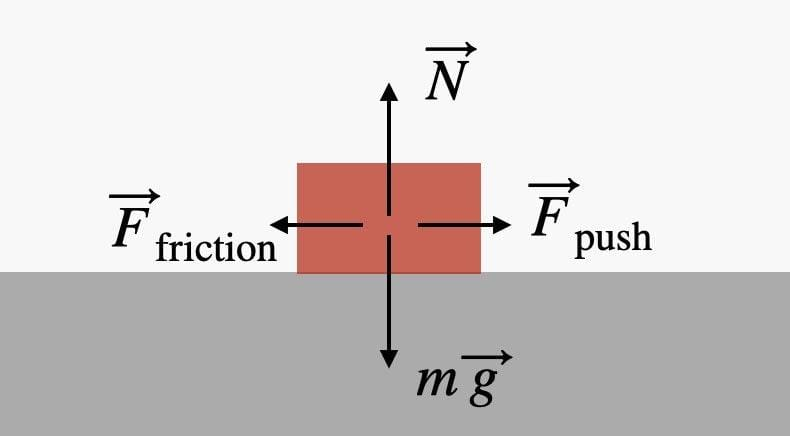Types of Friction

Before we proceed further into detailed account of frictional phenomena, it is advisable to become familiar with different types of frictional forces. All types of frictional phenomenon can be categorized into dry friction,fluid friction, and internal friction.

1. Dry Friction

It exists when two solid un-lubricated surfaces are in contact under the condition of sliding or tendency of sliding. It is also known as Coulomb friction.

➢  Types of Dry Friction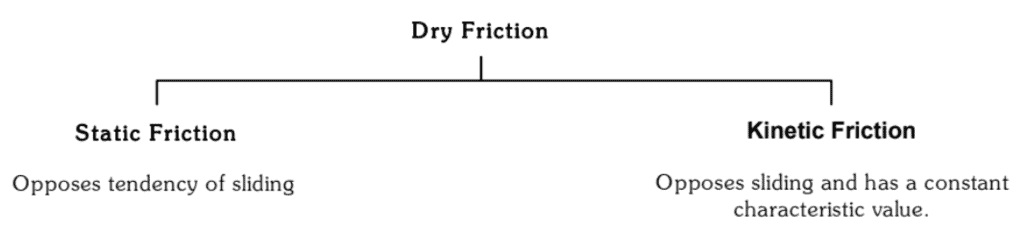In mechanics of non-deformable bodies, we are always concerned with the dry friction. Therefore, we often drop the word “dry” and simply call it friction.

To understand nature of friction let us consider a box of weight W placed on a horizontal rough surface.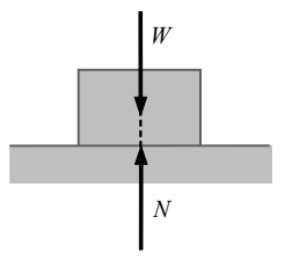The forces acting on the box are its weight and reaction from the horizontal surface. They are shown in the figure. The weight does not have any horizontal component, so the reaction of the horizontal surface on the box is normal to the surface. It is represented by N in the figure. The box is in equilibrium therefore both W and N are equal in magnitude, opposite in direction, and collinear. Now suppose the box is being pulled by a gradually increasing horizontal force F to slide the box.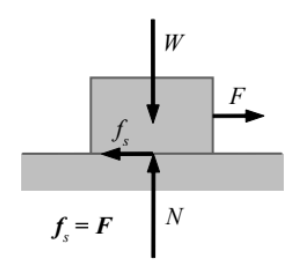Static Friction

Initially when the force F is small enough, the box does not slide. This can only be explained if we assume a frictional force, which is equal in magnitude and opposite in direction to the applied force F acts on the box. The force F produces in the box a tendency of sliding and the friction force is opposing this tendency of sliding. The frictional force developed before sliding initiates is defined as static friction. It opposes tendency of sliding.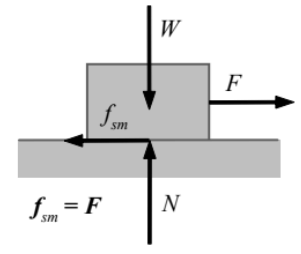Limiting Friction: The maximum Static Friction

As we increase F, the box remains stationary until a value of F is reached Kinetic friction when the box starts sliding. Before the box starts sliding, the static friction increases with F and counterbalances F until the static friction reaches its maximum value known as limiting friction or maximum static friction fsm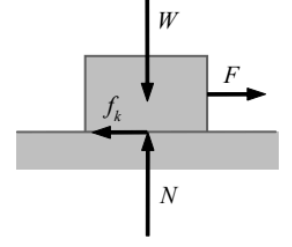Kinetic FrictionWhen the box starts sliding, to maintain it sliding still a force F is needed to over come frictional force. This frictional force is known as kinetic friction (fk ).It always opposes sliding.

2. Fluid Friction

Fluid friction is developed when adjacent layers of a fluid move at different velocities and gives birth to phenomena, which we call viscosity of the fluid. Resistance offered to motion of a solid body in a fluid also comes in this category and commonly known as viscous drag. We will study this kind of friction in fluid mechanics.

3. Internal Friction

When solid materials are subjected to deformation, internal resistive forces developed because of relative movement of different parts of the solid. These internal resistive forces constitute a system of force, which is defined as internal friction. They always cause loss of energy.

Frictional forces exist everywhere in nature and result in loss of energy that is primarily dissipated in form of heat. Wear and tear of moving bodies is another unwanted result of friction. Therefore, sometimes, we try to reduce their effects – such as in bearings of all types, between piston and the inner walls of the cylinder of an IC engine, flow of fluid in pipes, and aircraft and missile propulsion through air.

Though these examples create a negative picture of frictional forces, yet there are other situations where frictional forces become essential and we try to maximize the effects. It is the friction between our feet and the earth surface, which enables us to walk and run. Both the traction and braking of wheeled vehicles depend on friction.

Laws of Friction

When a normal force N exists between two surfaces, and we try to slide them over each other, the force of static friction (fs) acts before sliding initiates. It can have a value maximum up to the limiting friction (fsm).

fs ≤ fsm

The limiting friction is experimentally observed proportional to the normal reaction between surfaces in contact.

fsm = μsN

Here μs is the constant of proportionality. It is known as the coefficient of static friction for the two surfaces involved.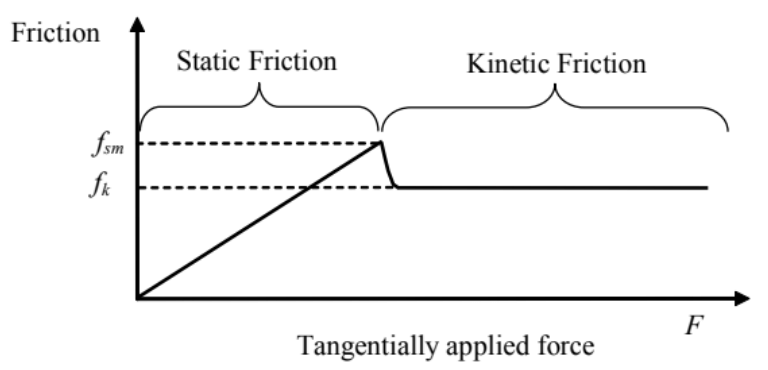When sliding starts between the surfaces, the frictional force rapidly drops to a characteristic value, which always opposes the sliding.

This characteristic frictional force is known as kinetic friction (fk). Kinetic friction is experimentally found proportional to the normal reaction between surfaces in contact.

fk = μkN

Here μis the constant of proportionality. It is known as the coefficient of kinetic friction for the two surfaces involved. The frictional forces between any pair of surfaces are decided by the respective coefficients of friction. The coefficients of friction are dimensionless constants and have no units. The coefficient of static fiction (μs) is generally larger than the coefficient of kinetic friction (μk) but never become smaller; at the most both of them may be equal. Therefore, the magnitude of kinetic friction is usually smaller than the limiting static friction (fsm).and sometimes kinetic friction becomes equal to the limiting static friction but it can never exceed the limiting friction.

Note:

The limiting static friction and the kinetic friction between any pair of solid surfaces follow these two empirical laws:

(i) Frictional forces are independent of measured area of contact.

(ii) Both the limiting static friction and kinetic friction are proportional to the normal force pressing the surfaces in contact.

Angle of Friction

The angle of friction is the angle between resultant contact force of and normal reaction N, when sliding is initiating. It is denoted by λ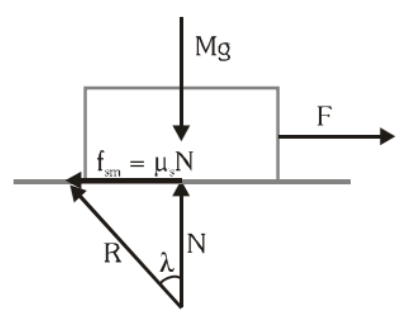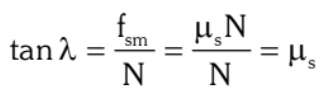For smooth surface λ = 0

Angle of Repose (θ)

A body is placed on an inclined plane and the angle of inclination is gradually increased. At some angle of inclination θ the body starts sliding down the plane due to gravity. This angle of inclination is called angle of repose (θ).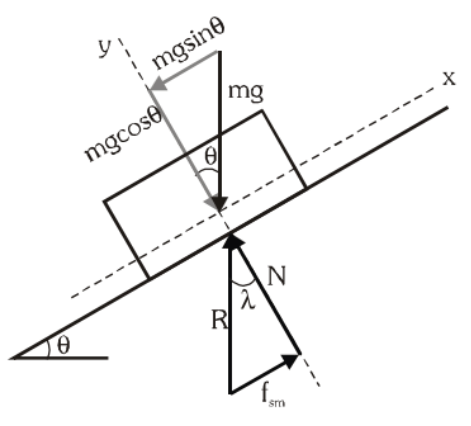Angle of repose is that minimum angle of inclination at which a body placed on the inclined starts sliding down due to its own weight. Thus, angle of repose = angle of friction.

➤ Example.1. A block of mass 1 kg is at rest on a rough horizontal surface, where coefficients of static and kinetic friction are 0.2 and 0.15.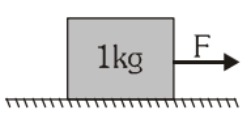Find the frictional forces if a horizontal force

(a) F = 1N (b) F = 1.96 N (c) F = 2.5 N is applied on a block

Solution. Maximum force of friction is the limiting friction fsm = 0.2 × 1 × 9.8 N = 1.96 N

(a) For F = 1 N, F < fsm

So, body is in rest means static friction is present and hence fs = F = 1 N

(b) For F = 1.96 N, F = fsm = 1.96 N. The block is about to slide, therefore f = 1.96 N

(c) For F = 2.5 N, So F > fsm

Now body is sliding and kinetic friction acts.

Therefore f = fk =  μkN =  μmg = 0.15 × 1 × 9.8 = 1.47 N

➤ Example.2. Length of a uniform chain is L and coefficient of static friction is μ between the chain and the table top. Calculate the maximum length of the chain which can hang from the table without sliding.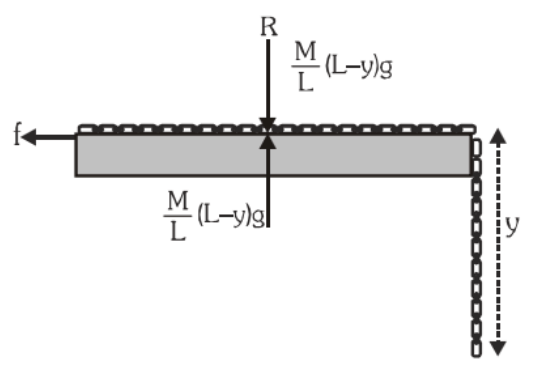Solution. Let y be the maximum length of the chain that can hang without causing the portion of chain on table to slide.

Length of chain on the table = (L – y)

Weight of part of the chain on table =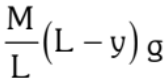Weight of hanging part of the chain =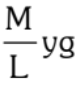For equilibrium with maximum portion hanging,

limiting friction = weight of hanging part of the chain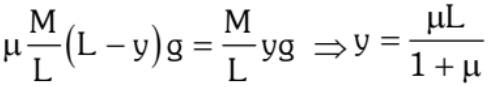Motion on a Rough Inclined Plane

Suppose a motion up the plane takes place under the action of pull P acting parallel to the plane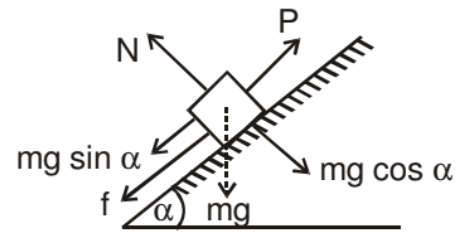N = mg cos α
Frictional force acting down the plane F - μN = μ mg cos α
Appling Newton's second law for motion up the plane
P - (mg sin α + f) = ma
P - mg sin α - μ mg cos α - ma
If P = 0 the block may slide downwards with an acceleration a.
The frictional force would then act up the plane
mg sin α - F = ma
mg sin α - μ mg cos α = ma

➤ Example.3. A 20 kg box is gently placed on a rough inclined plane of inclination 30° with horizontal. The coefficient of sliding friction between the box and the plane is 0.4. Find the acceleration of the box down the incline.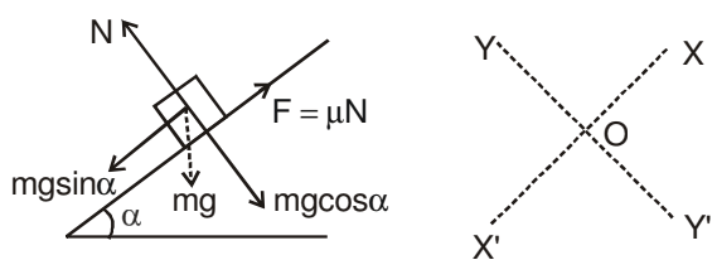Solution. In solving inclined plane problems, the X and Y directions along which the forces are to be considered, may be taken as shown. The components of weight of the box are

(i) mg sin α acting down the plane and
(ii) mg cos α acting perpendicular to the plane.
N = mg cos α
mg sin α - μ N = ma ⇒ mg sin α - μ mg cos α = ma
a = g sin α - μg cos α

a = g (sin α - μ cos α)

a =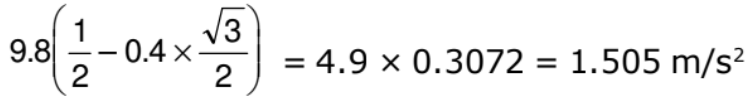The box accelerates down the plane at 1.505 m/s2.

➤ Example.4. A force of 400 N acting horizontal pushes up a 20 kg block placed on a rough inclined plane which makes an anlge of 45° with the horizontal. The acceleration experienced by the block is 0.6 m/s2. Find the coefficient of sliding friction between the box and incline.

Solution. The horizontally directed force 400 N and weight 20 kg of the block are resolved into two mutually perpendicular components, parallel and perpendicular to the plane as shown.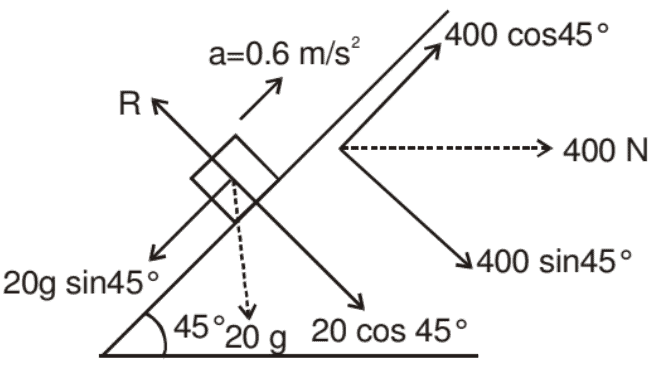N = 20 g cos 45° + 400 sin 45° = 421.4 N

The frictional force experienced by the block

F = μN = μ x 421.4 = 421.4 μN.

As the accelerated motion is taking placed up the plane.

400 cos 45° - 20 g sin 45° - f = 20a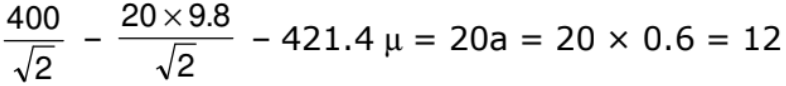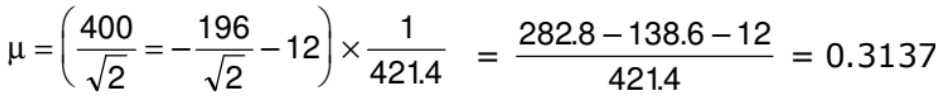The coefficient of sliding friction between the block and the incline = 0.3137

Two Blocks on an Inclined Plane

Consider two blocks having masses m1 & m2 placed on a rough inclined plane. μ1 & μ2 are the friction coefficient for m1 & m2 respectively. If N is the normal force between the contact surface of m1 & m2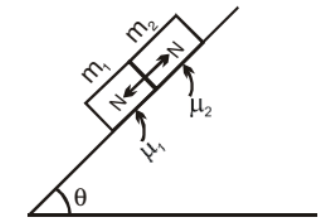Now three condition arises:

(i) If μ1 = μ2= μ then
N = 0 because, Both the blocks are in contact but does not press each other.

a1 = a2 = g sin θ - μ mg cos θ
(a1, a2 are acceleration of block μ1 & μ2 respectively)

(ii) If μ1 < μ2 then

N = 0 because, there is no contact between the blocks.

a1 = g sin θ - μ1 g cos θ
a2 = g sin θ - μ2 cos θ

⇒ a1 > a2

(iii)  If μ1 > μ2 then    N ≠ 0
a1 = a2

➤ Example.5. Mass m1 & m2 are placed on a rough inclined plane as shown in figure. Find out the acceleration of the blocks and contact force in between these surface.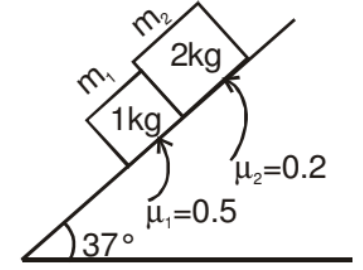Sol. As we know if μ1 > μ2 both will travel together so

a1 = a2 = a

F.B.D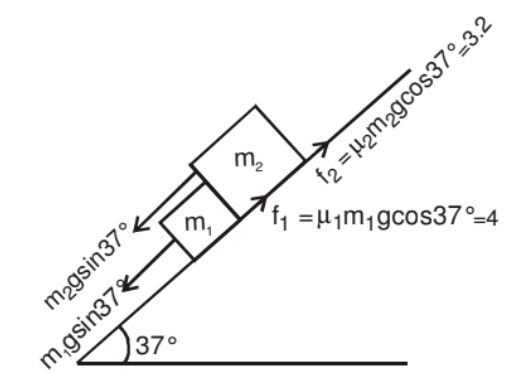which is equivalent to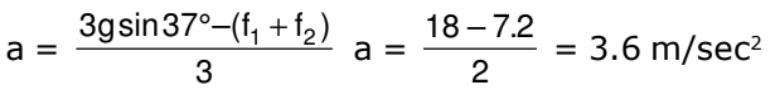Now F.B.D of 1 kg block is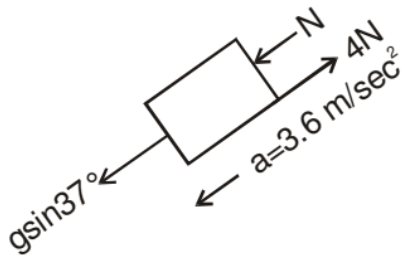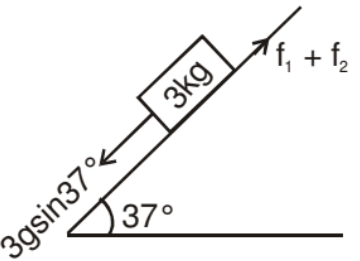gsin37° + N - 4 = (1) a

N = 3.6 +  4 - 6 = 1.6 Newton

Pulley Block System Involving Friction

If friction force is acting and value of acceleration of a particle is negative, then it means direction of friction force is opposite to that what we assumed and acceleration would be having a different numerical value.

➤ Example.6. Two blocks of masses 5 kg and 10 kg are attached with the help of light string and placed on a rough incline as shown in the figure. Coefficients of friction are as marked in the figure. The system is released from rest. Determine the acceleration of the two blocks.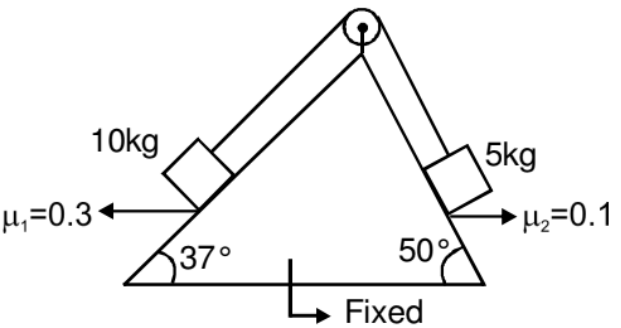Solution. Let 10 kg block is sliding down, then acceleration of both the blocks are given by,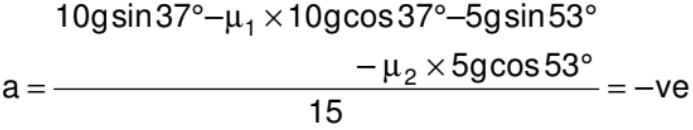It means our assumed direction of motion is wrong and 5 kg block is going to slide down, if this would be the case, the direction of friction force will reverse and acceleration of blocks would be given by :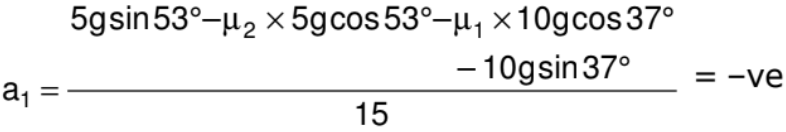It means in this direction also there is no motion. So we can conclude that the system remains at rest and friction force is static in nature.

Two Block System

➤ Example.7. Find out the maximum value of F for which both the blocks will move together.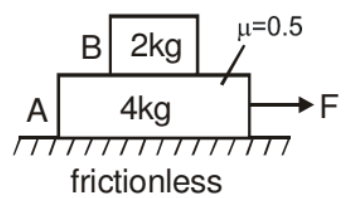Solution. In the given situation 2kg block will move only due to friction force exerted by the 4 kg block

F.B.D.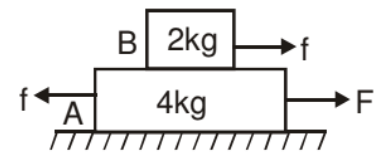The maximum friction force exerted on the block B is

fmax = μN

fmax = (0.5) (20) = 10 N

So the maximum acceleration of 2 kg block is

a2max = 10/2 = 5 m/s2

amax is the maximum acceleration for which both the block will move together. i.e., for a £ 5 ms-2 acceleration of both blocks will be same and we can take both the blocks as a system.

F.B.D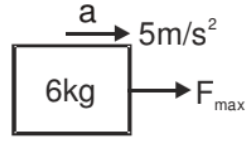Fmax = 6 × 5 = 30 N

for 0 < F < 30

Both the block move together.

➤ Example.8. In the above question find the acceleration of both the block when

(i) F = 18 N

(ii) F = 36 N

Solution. (i) Since F < 30 both the blocks will move together

F.B.D.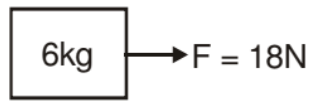(ii) When F = 36 N

When F > 30 both the blocks will move separately so we treat each block independently

F.B.D of 2 kg block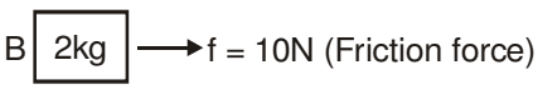aB = 5 m/s2

F.B.D of 4 kg block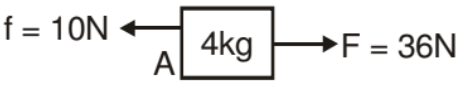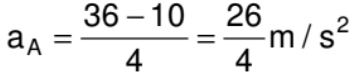➤ Example.9. Find out the range of force in which both the blocks move together.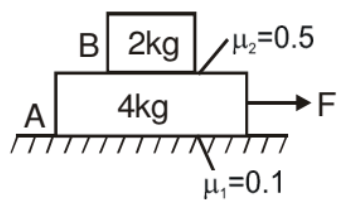Solution. If f1 is friction force between block A & lower surface and f2 is friction force between both the block's surface.

F.B.D.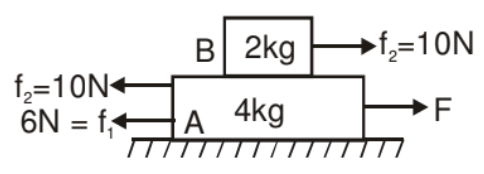f1 max = m1N1 = (0.1) (60) = 6 N

f2 max = m2N2 = (0.5) (20) = 10 N

Upper 2kg block is move only due to friction force so maximum acceleration of that block is

amax = 10/2 = 5m/s2

This is the maximum acceleration for which both the blocks will move together.

Therefore for a ≤ 5ms-2 we can take both the blocks as one system.

F.B.D.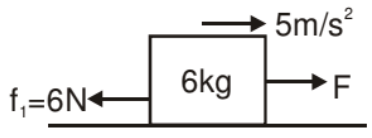For F < 6 N. Blocks will not move at all.

Now the value of Fmax for which both the blocks will move together.

Fmax - 6 = 6 × 5

Fmax = 36 N

Conclusion if

0N < F < 6N No blocks will move

6N < F < 36 N Both blocks will move together

F > 36 N Both move separately.

Friction Involving Pseudo Concept

➤ Example.10. What is the minimum acceleration with which bar A should be shifted horizontally to keep the bodies 1 and 2 stationary relative to the bar ? The masses of the bodies are equal and the coefficient of friction between the bar and the bodies equal to m. The masses of the pulley and the threads are negligible while the friction in the pulley is absent. see in fig.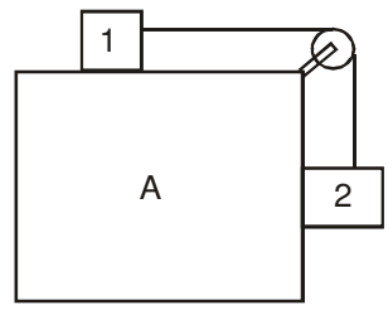Solution. Let us place the observer on A.

Since we have non-inertial frame we have pseudo forces.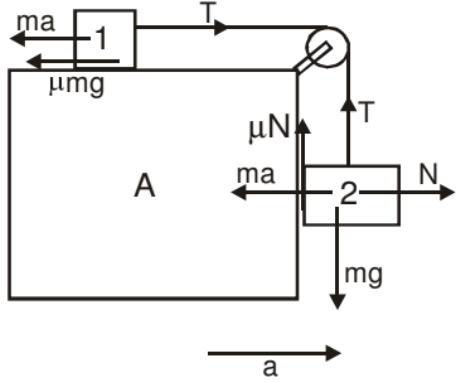For body '1' we have,

T = ma + μmg ....(1)

For body '2' we have,

N = ma

mg - T - μma = 0

mg = T + μmg ....(2)

From (1) and (2)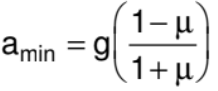➤ Example.11. Mass m2 placed on a plank of mass m1 lying on a smooth horizontal plane. A horizontal force F = a0t (a0 is a constant) is applied to a bar. If acceleration of the plank and bar are a1 and a2 respectively and the coefficient of friction between m1 and m2 is m. Then find acceleration a with time t.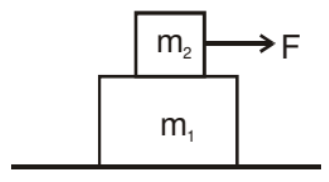Sol. If F < mm2g then both blocks move with common acceleration, i.e., a1 = a2

When F > μm2g, then

Equation for block of mass m

F - μm2g = m2a2      ...(1)

μm2g = m1a1          ...(2)

From equation (1)

αοt - μm2g = m2a2

i.e., acceleration a2 varies with time linearly, its slope positive and intercept negative.

From equation (2) a1 is independent of time.

So, the graph between a & t is as follow.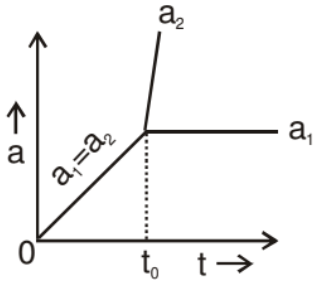The document Friction & Its Types - Notes | Study Physics Class 11 - NEET is a part of the NEET Course Physics Class 11.
All you need of NEET at this link: NEET

## Physics Class 11

127 videos|464 docs|210 tests

## Physics Class 11

127 videos|464 docs|210 tests

### How to Prepare for NEET

Read our guide to prepare for NEET which is created by Toppers & the best Teachers

Track your progress, build streaks, highlight & save important lessons and more!

,

,

,

,

,

,

,

,

,

,

,

,

,

,

,

,

,

,

,

,

,

;